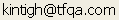Tools for Quantitative Archaeology    Email:TRIANGLE: Format Triangular Matrix for Printing

Program triangle is a simple utility that reads a diagonal-absent triangular matrix of the form produced by DISTANCE (and various Antana programs) and formats and labels it in a way that is easy to read. It can read an upper triangular matrix with up to 148 observations. If there are N cases, the program expects to find N*(N-1)/2 similarity or distance measures. It formats the matrix for a user-specified page width with a user-specified number of decimals printed. Because it assumes that similarities or distances will generally be scaled to a range of -1 to 1, as they are by the DISTANCE program, it assumes that there are no more than one digit (with an possible minus sign) to the left of the decimal.

PROGRAM OPERATION

To start the program type: TRIANGLE <Enter>.The program prompts, shown in the sample interactive session, listed below, are self explanatory.

Upper Triangular Matrix Input File {.DST} ?

Name of triangular file, in Antana format. Triangular matrices in the format required here have as their first two values the number of cases and -1, indicating an upper triangular matrix.

Read Case Label File {N} ? Y

Case Label File Name {.ARL} ? con

Output File Name {.LST} ?

Set Output Options {N} ?

Print Matrix in [T]riangular or [S]quare Form {T} ? T

Matrices, by default, are printed in lower triangular form.

Output Line Width (in characters) {80} ?

Print Width of Observation Labels {6} ?

These affect output matrix spacing and labeling. A shorter width than the labels actually have can be specified to compress the matrix.

Scale Factor (Multiple Values by) {1} ?

Tables scaled 0-1 are easier to read if you multiply everything by 100, so values range from 0 to 100; decimal places can often be ignored.

Number of Decimal Places to Print {2} ?

After scaling, the number of decimals rounded to. For a scale factor of 100, there is a lot to gain in readability by rounding to 0 decimals, i.e. integers.

Program End

SAMPLE PROGRAM INPUT

```4 -1 #test matrix#
0.01  1.00  .95  1.00 .05  0.5```

PROGRAM OUTPUT

```Case B:   0.01
Case C:   1.00   1.00
Case D:   0.95   0.05   0.50
Case A Case B Case C```

 Home Top Overview Ordering Documentation

Page Last Updated - 02-Jun-2007